# Selina Solutions Concise Maths Class 10 Chapter 24 Measures of Central Tendency Exercise 24(E)

Recalling the concepts learnt from the previous chapter, this exercise contains a mixture of problems based on finding the mean, median, mode and drawing ogives for suitable questions. If students are searching for a resource to clarify their doubts, then stop here at the Selina Solutions for Class 10 Maths as these solutions are created by experts at BYJU’S, keeping in mind the latest ICSE patterns. These exercise solutions are available in the Concise Selina Solutions for Class 10 Maths Chapter 24 Measures of Central Tendency Exercise 24(E) PDF, provided in the link mentioned below.

## Selina Solutions Concise Maths Class 10 Chapter 24 Measures of Central Tendency Exercise 24(E) Download PDF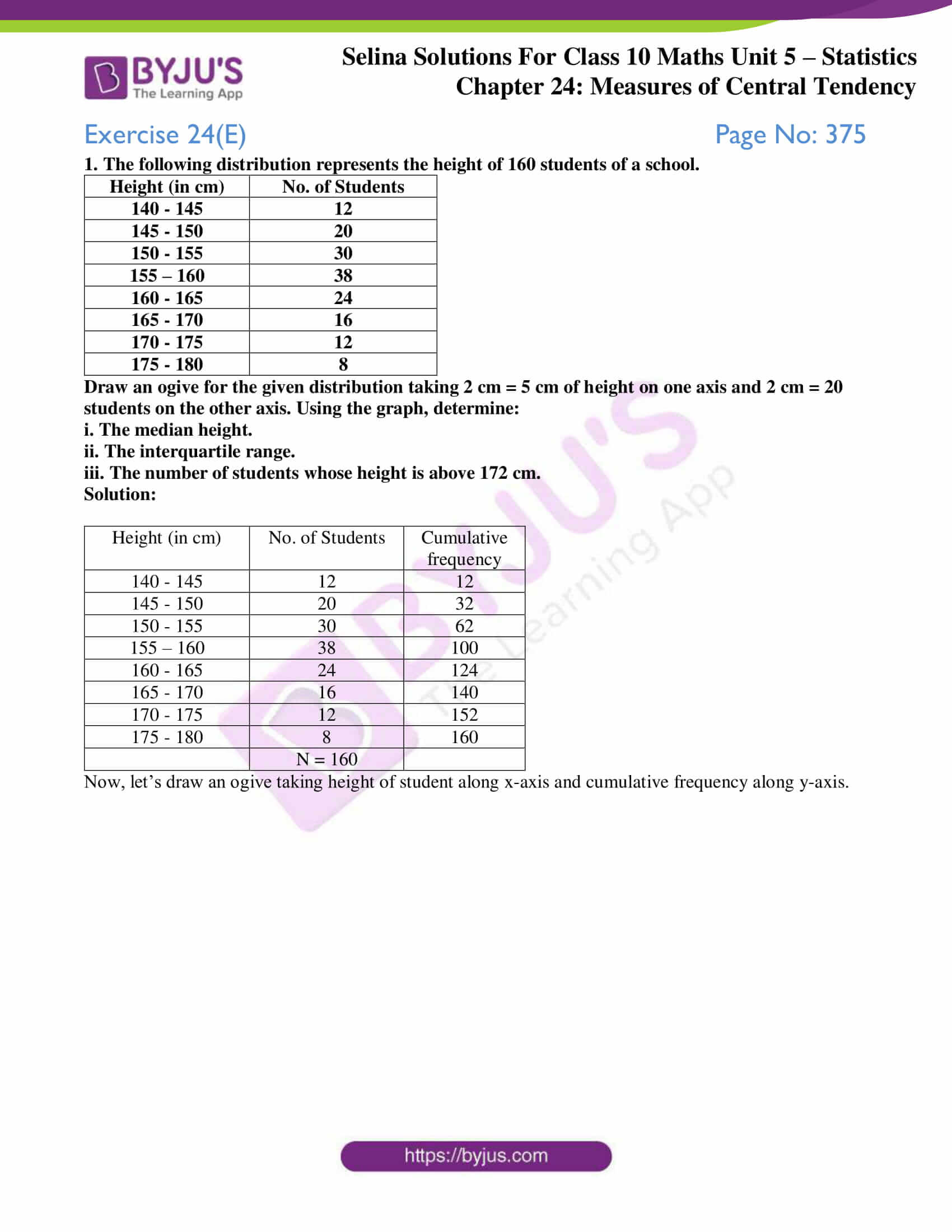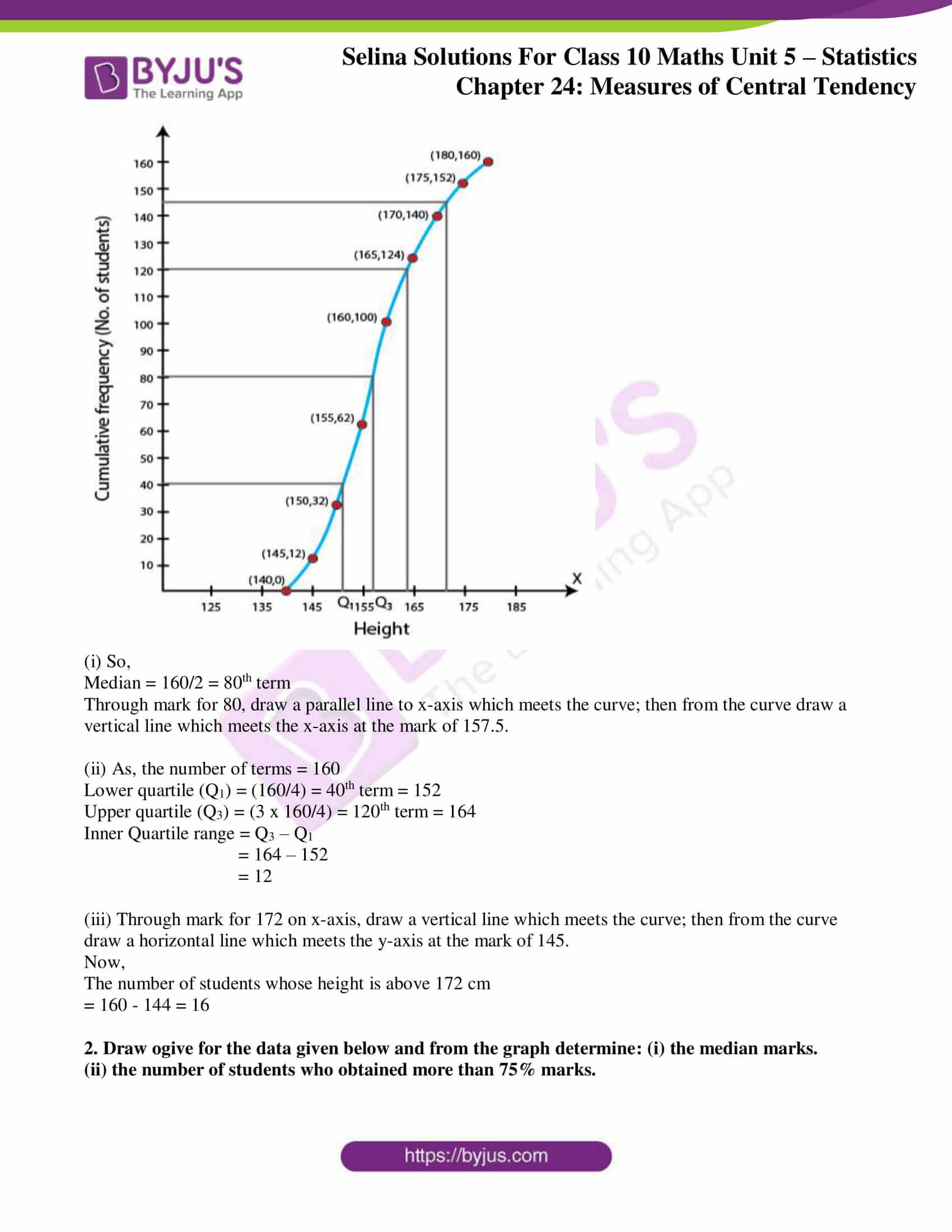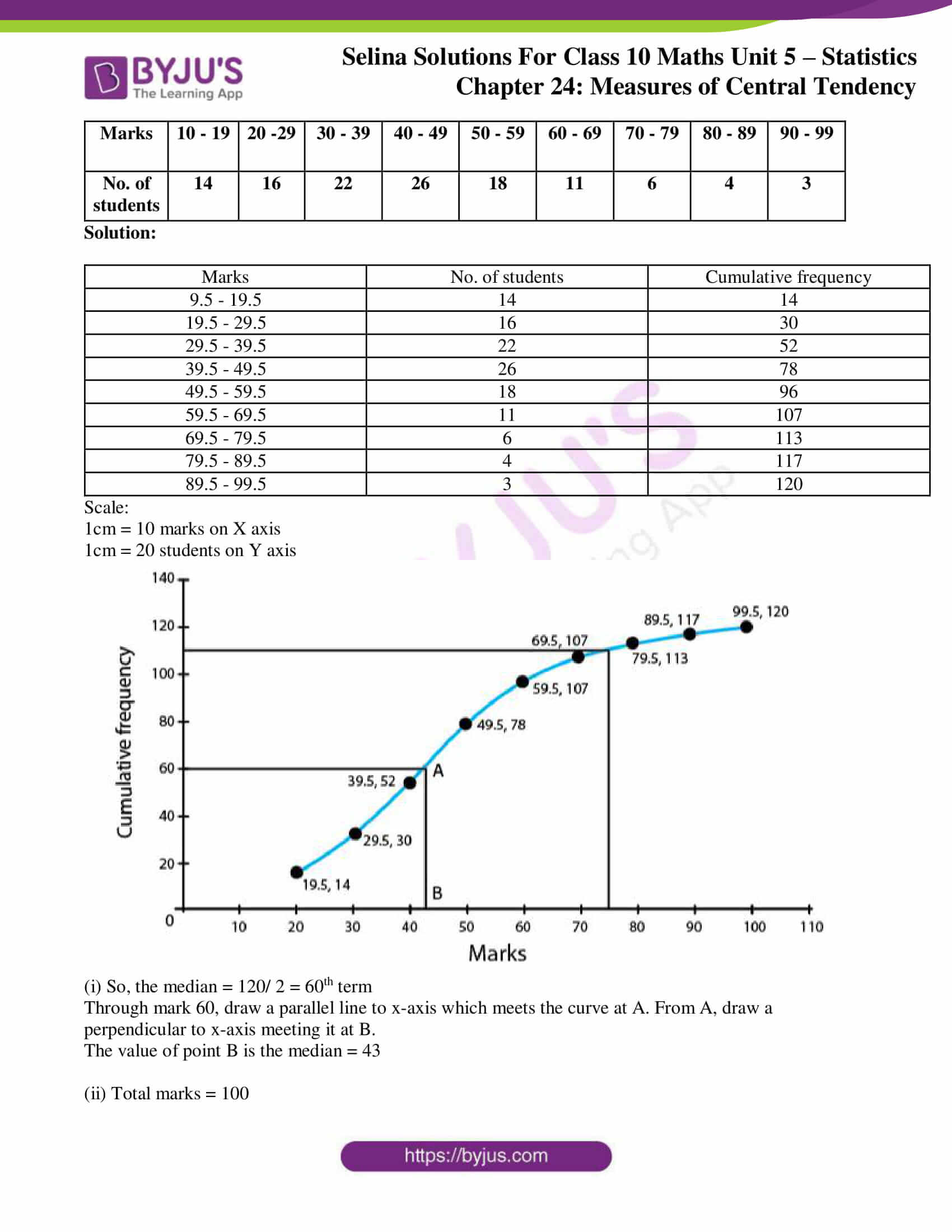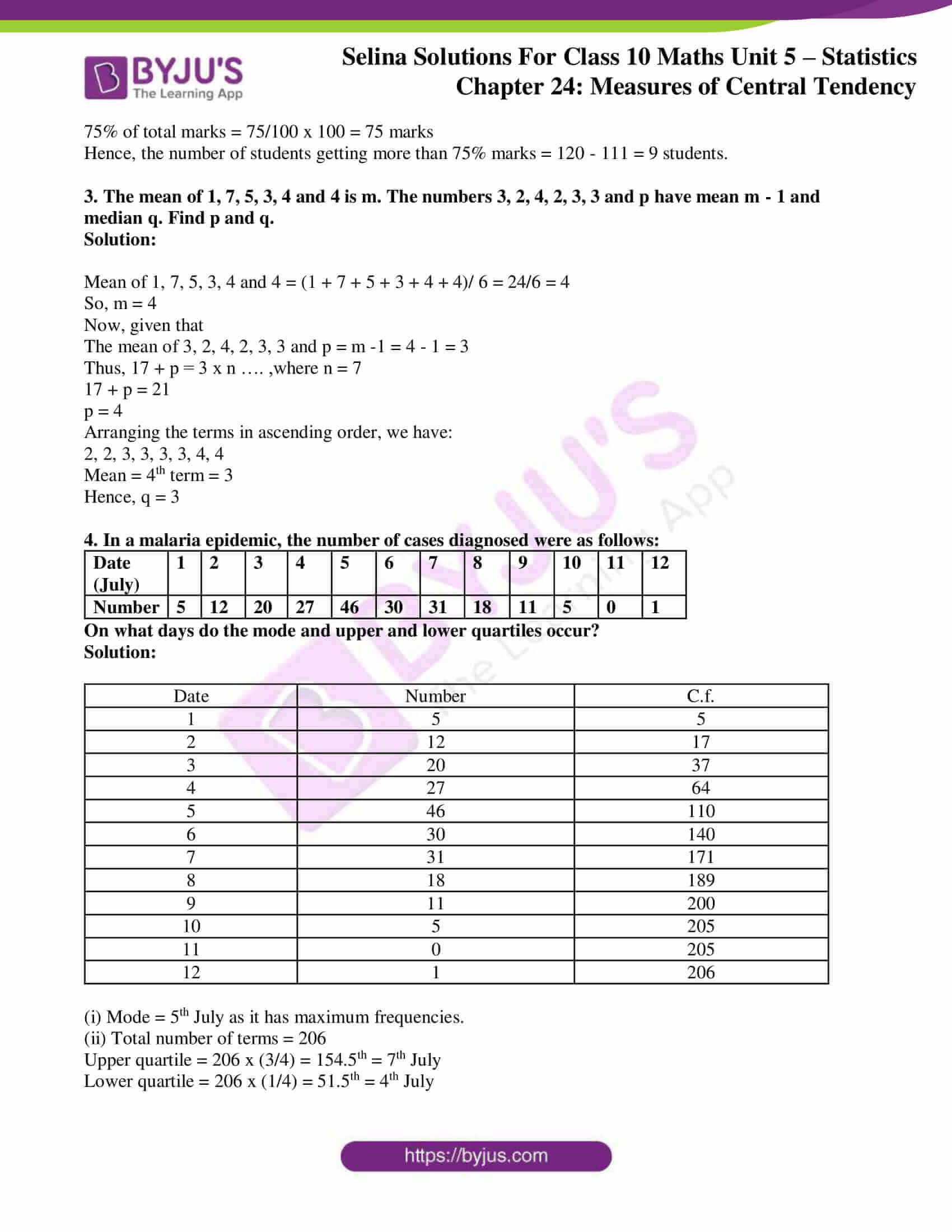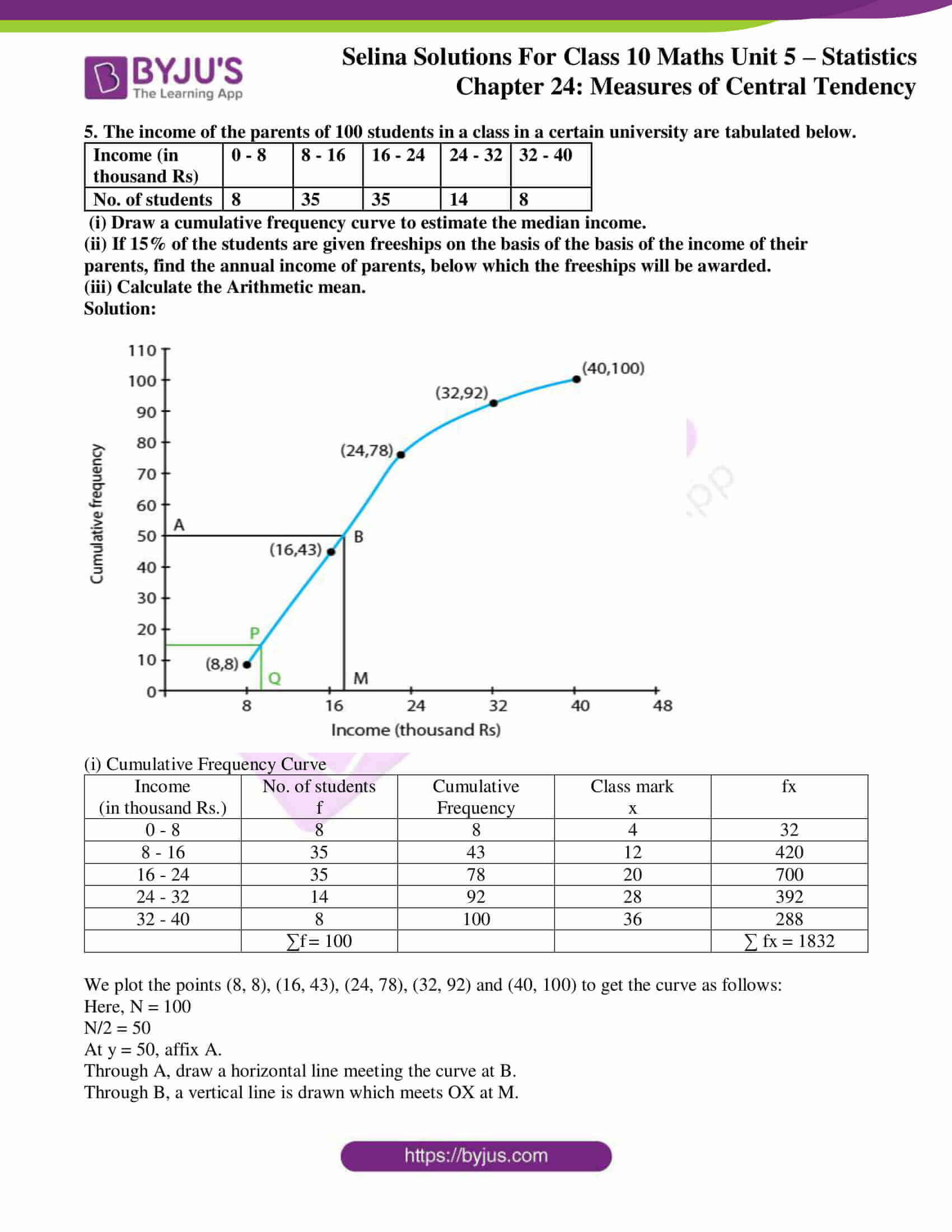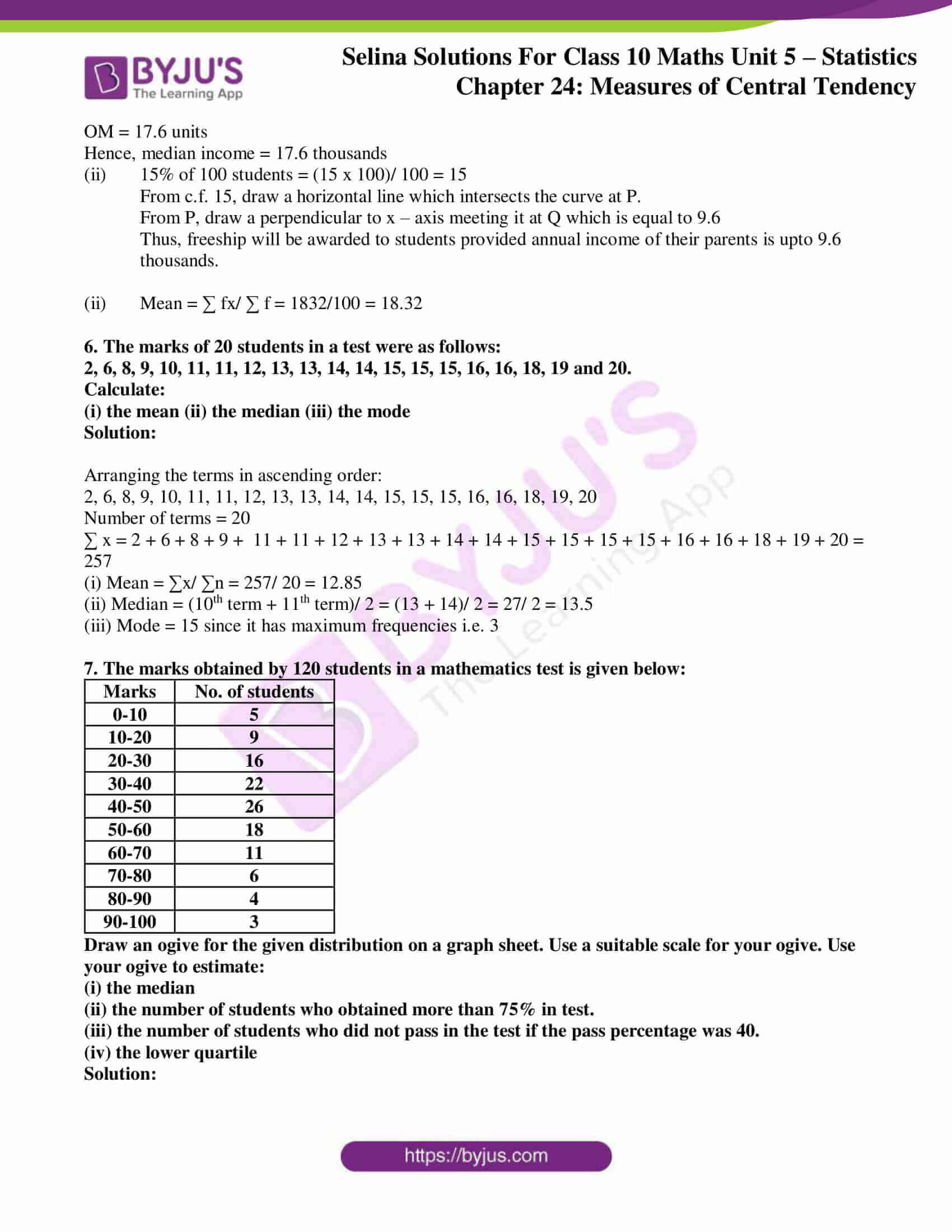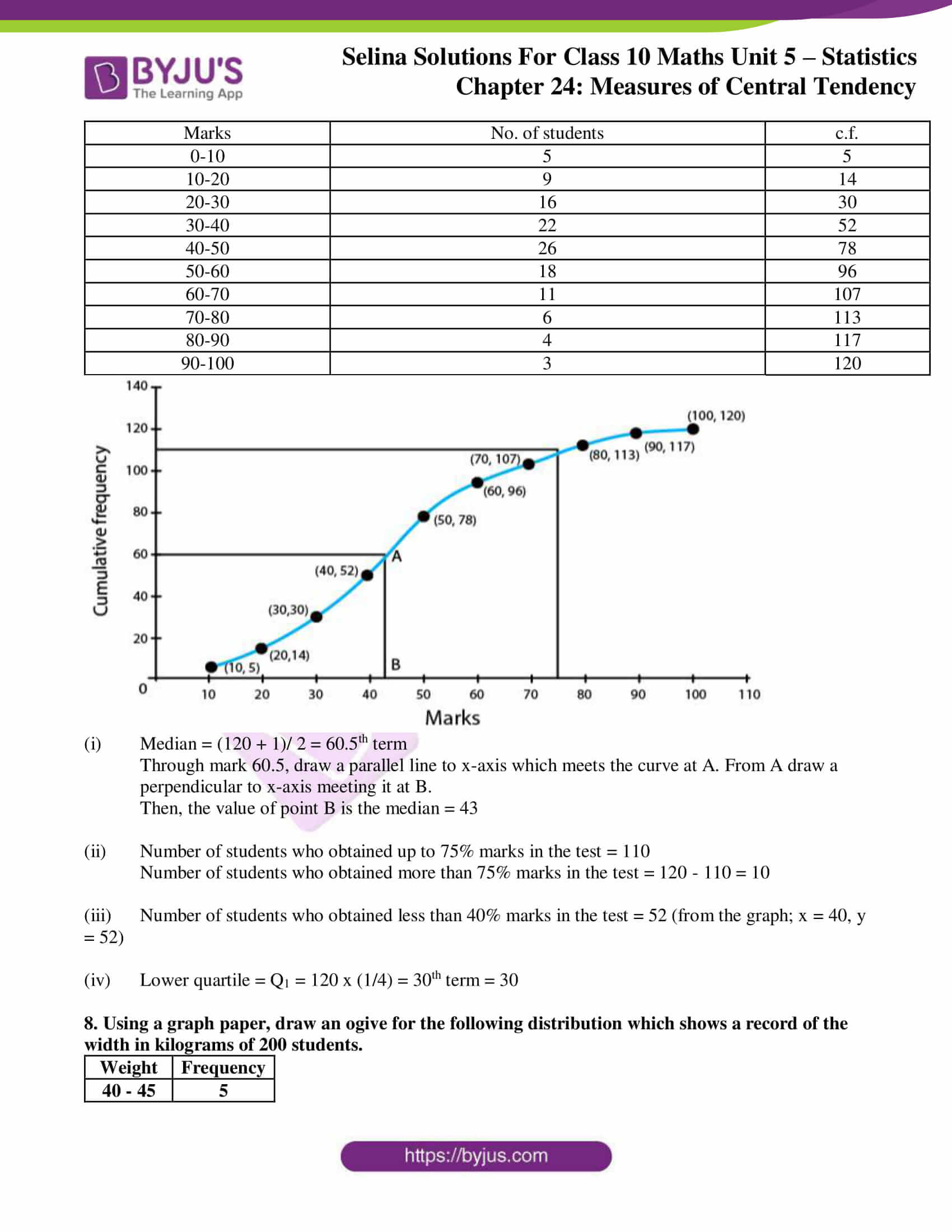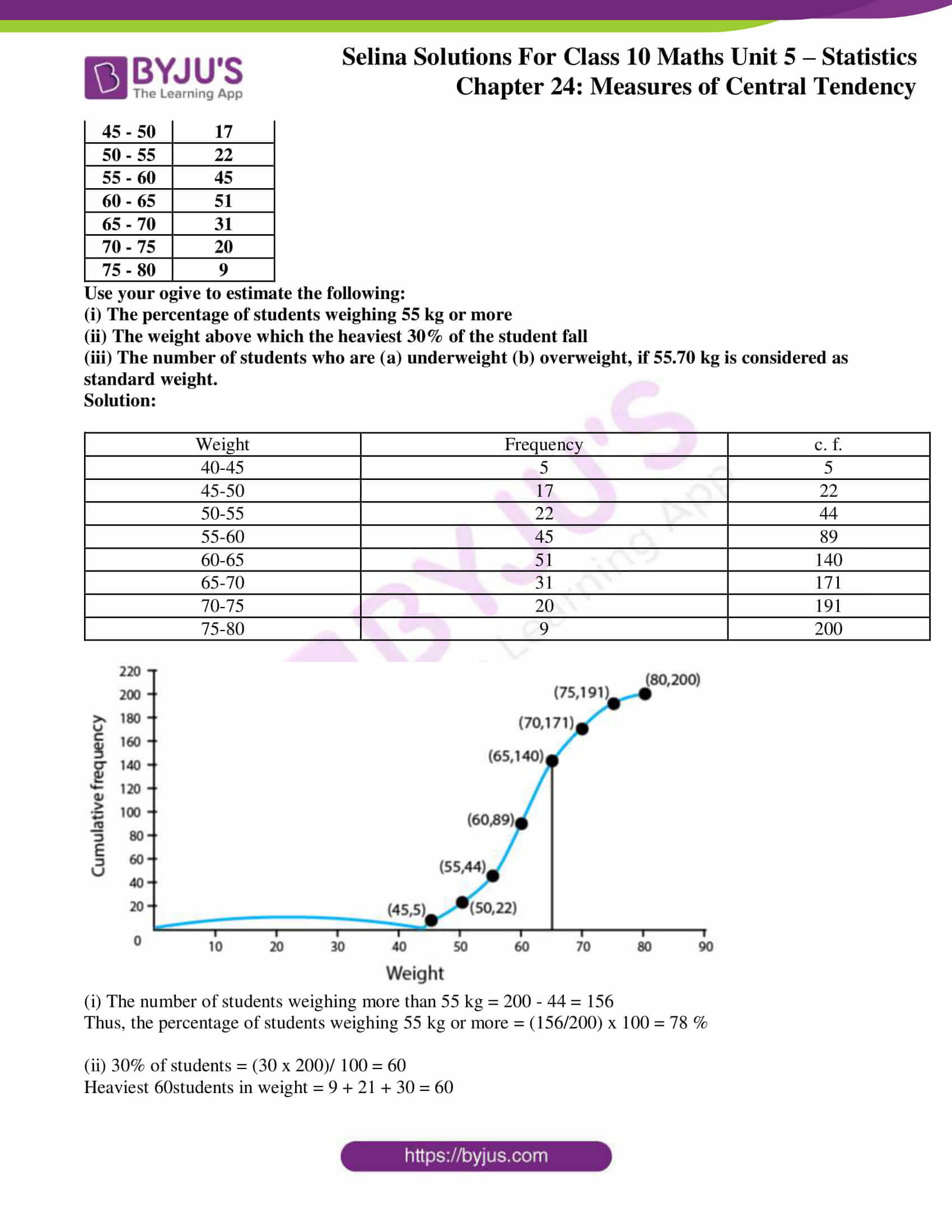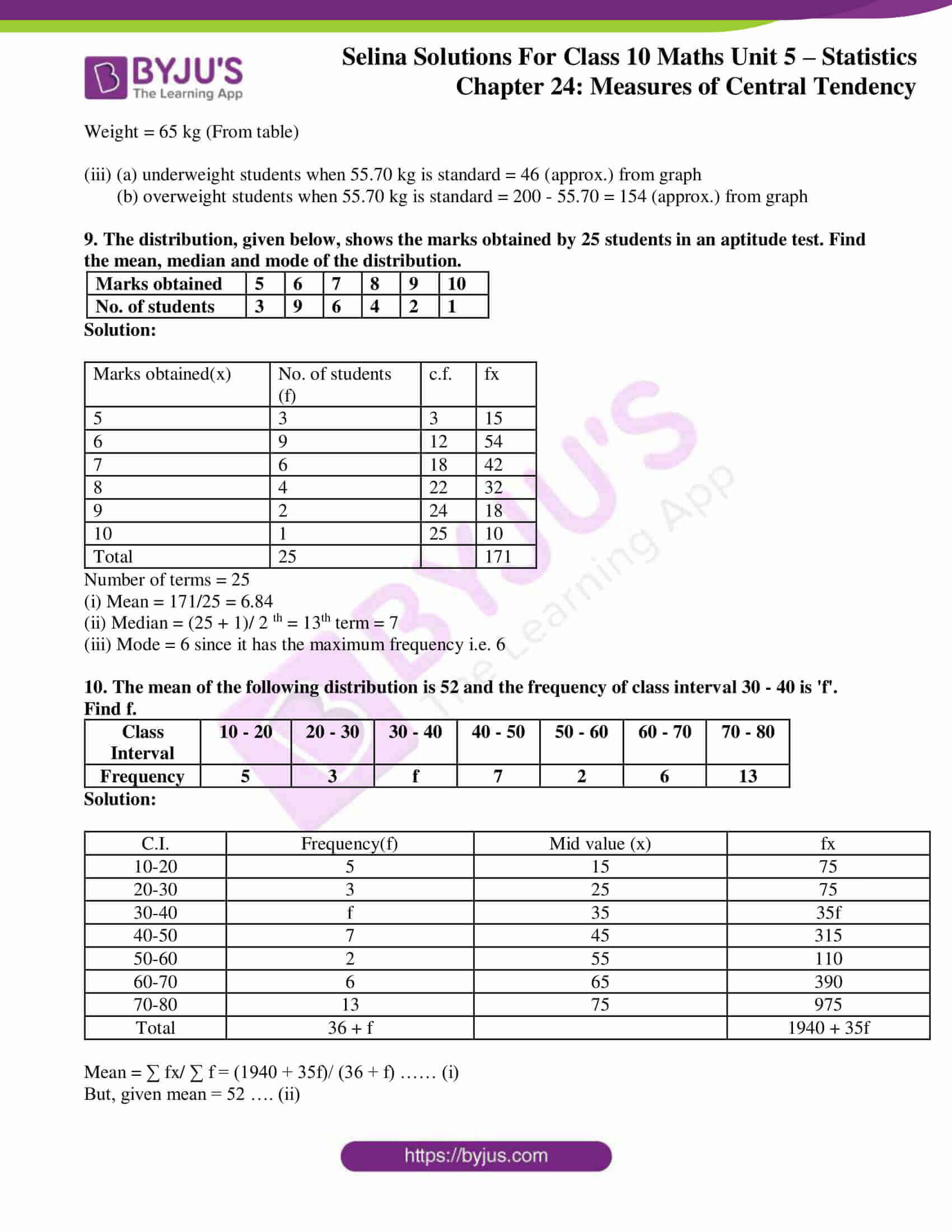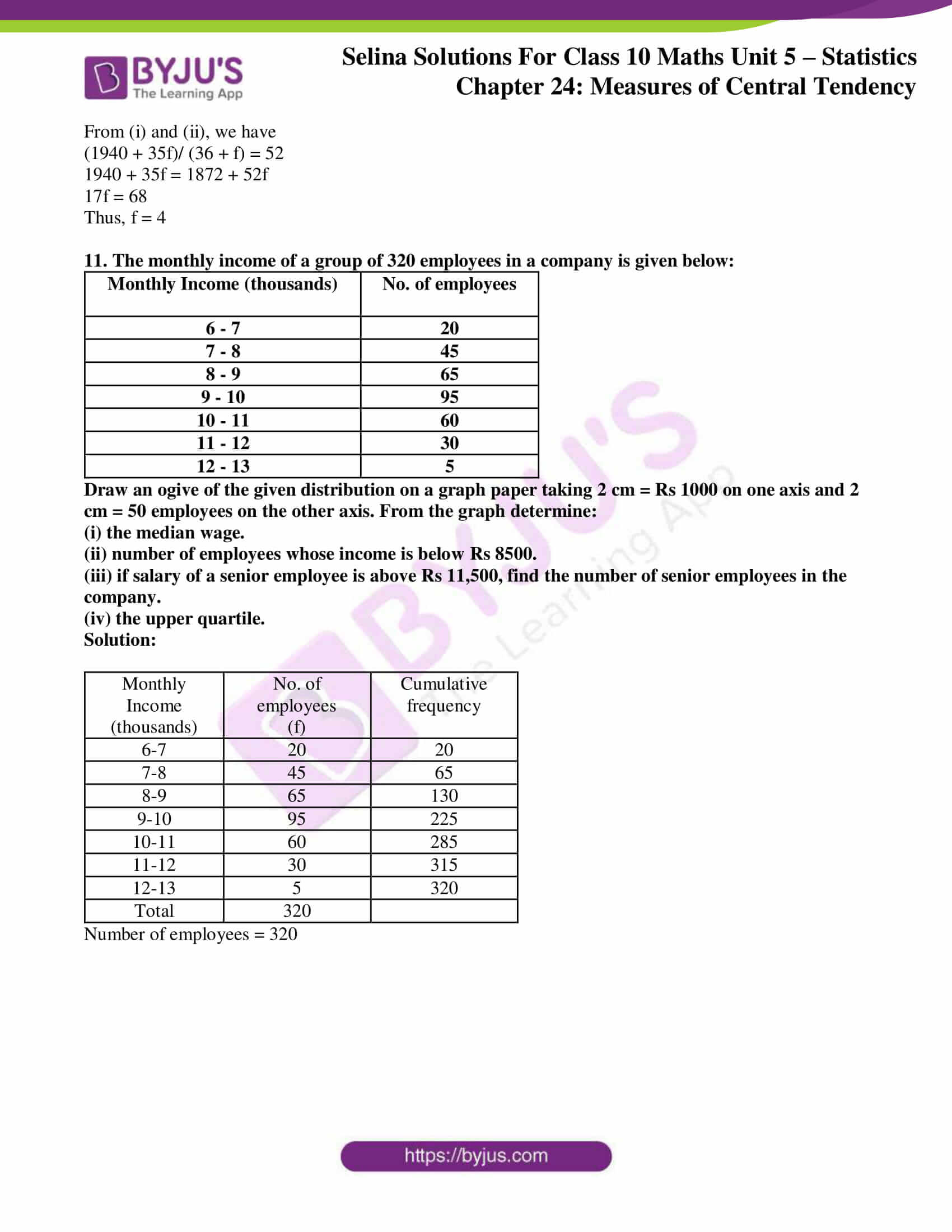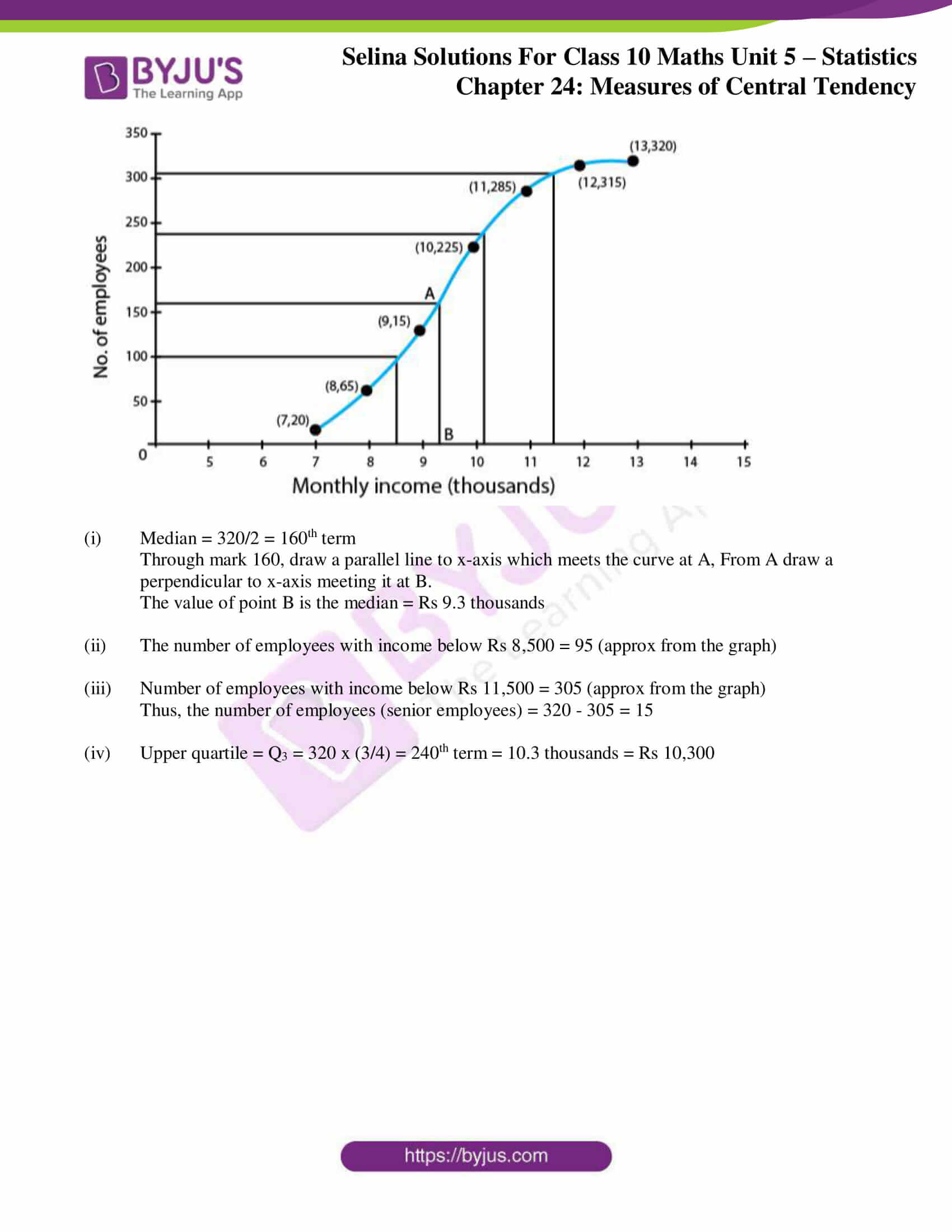### Access Selina Solutions Concise Maths Class 10 Chapter 24 Measures of Central Tendency Exercise 24(E)

1. The following distribution represents the height of 160 students of a school.

 Height (in cm) No. of Students 140 – 145 12 145 – 150 20 150 – 155 30 155 – 160 38 160 – 165 24 165 – 170 16 170 – 175 12 175 – 180 8

Draw an ogive for the given distribution taking 2 cm = 5 cm of height on one axis and 2 cm = 20 students on the other axis. Using the graph, determine:

i. The median height.

ii. The interquartile range.

iii. The number of students whose height is above 172 cm.

Solution:

 Height (in cm) No. of Students Cumulative frequency 140 – 145 12 12 145 – 150 20 32 150 – 155 30 62 155 – 160 38 100 160 – 165 24 124 165 – 170 16 140 170 – 175 12 152 175 – 180 8 160 N = 160

Now, let’s draw an ogive taking height of student along x-axis and cumulative frequency along y-axis.(i) So,

Median = 160/2 = 80th term

Through mark for 80, draw a parallel line to x-axis which meets the curve; then from the curve draw a vertical line which meets the x-axis at the mark of 157.5.

(ii) As, the number of terms = 160

Lower quartile (Q1) = (160/4) = 40th term = 152

Upper quartile (Q3) = (3 x 160/4) = 120th term = 164

Inner Quartile range = Q3 – Q1

= 164 – 152

= 12

(iii) Through mark for 172 on x-axis, draw a vertical line which meets the curve; then from the curve draw a horizontal line which meets the y-axis at the mark of 145.

Now,

The number of students whose height is above 172 cm

= 160 – 144 = 16

2. Draw ogive for the data given below and from the graph determine: (i) the median marks.

(ii) the number of students who obtained more than 75% marks.

 Marks 10 – 19 20 -29 30 – 39 40 – 49 50 – 59 60 – 69 70 – 79 80 – 89 90 – 99 No. of students 14 16 22 26 18 11 6 4 3

Solution:

 Marks No. of students Cumulative frequency 9.5 – 19.5 14 14 19.5 – 29.5 16 30 29.5 – 39.5 22 52 39.5 – 49.5 26 78 49.5 – 59.5 18 96 59.5 – 69.5 11 107 69.5 – 79.5 6 113 79.5 – 89.5 4 117 89.5 – 99.5 3 120

Scale:

1cm = 10 marks on X axis

1cm = 20 students on Y axis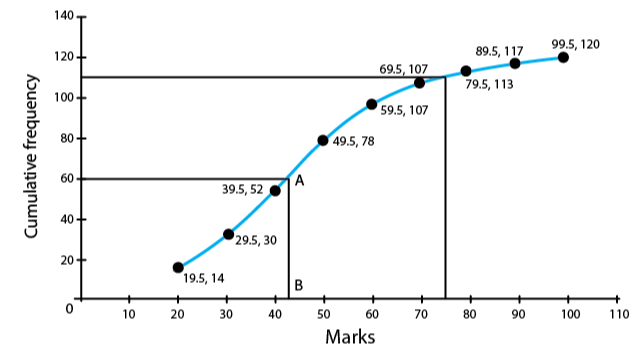(i) So, the median = 120/ 2 = 60th term

Through mark 60, draw a parallel line to x-axis which meets the curve at A. From A, draw a perpendicular to x-axis meeting it at B.

The value of point B is the median = 43

(ii) Total marks = 100

75% of total marks = 75/100 x 100 = 75 marks

Hence, the number of students getting more than 75% marks = 120 – 111 = 9 students.

3. The mean of 1, 7, 5, 3, 4 and 4 is m. The numbers 3, 2, 4, 2, 3, 3 and p have mean m – 1 and median q. Find p and q.

Solution:

Mean of 1, 7, 5, 3, 4 and 4 = (1 + 7 + 5 + 3 + 4 + 4)/ 6 = 24/6 = 4

So, m = 4

Now, given that

The mean of 3, 2, 4, 2, 3, 3 and p = m -1 = 4 – 1 = 3

Thus, 17 + p = 3 x n …. ,where n = 7

17 + p = 21

p = 4

Arranging the terms in ascending order, we have:

2, 2, 3, 3, 3, 3, 4, 4

Mean = 4th term = 3

Hence, q = 3

4. In a malaria epidemic, the number of cases diagnosed were as follows:

 Date (July) 1 2 3 4 5 6 7 8 9 10 11 12 Number 5 12 20 27 46 30 31 18 11 5 0 1

On what days do the mode and upper and lower quartiles occur?

Solution:

 Date Number C.f. 1 5 5 2 12 17 3 20 37 4 27 64 5 46 110 6 30 140 7 31 171 8 18 189 9 11 200 10 5 205 11 0 205 12 1 206

(i) Mode = 5th July as it has maximum frequencies.

(ii) Total number of terms = 206

Upper quartile = 206 x (3/4) = 154.5th = 7th July

Lower quartile = 206 x (1/4) = 51.5th = 4th July

5. The income of the parents of 100 students in a class in a certain university are tabulated below.

 Income (in thousand Rs) 0 – 8 8 – 16 16 – 24 24 – 32 32 – 40 No. of students 8 35 35 14 8

(i) Draw a cumulative frequency curve to estimate the median income.

(ii) If 15% of the students are given freeships on the basis of the basis of the income of their parents, find the annual income of parents, below which the freeships will be awarded.

(iii) Calculate the Arithmetic mean.

Solution: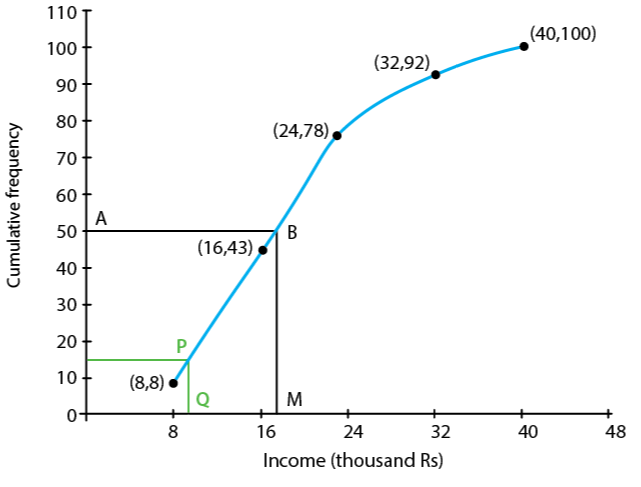(i) Cumulative Frequency Curve

 Income (in thousand Rs.) No. of students f Cumulative Frequency Class mark x fx 0 – 8 8 8 4 32 8 – 16 35 43 12 420 16 – 24 35 78 20 700 24 – 32 14 92 28 392 32 – 40 8 100 36 288 ∑f = 100 ∑ fx = 1832

We plot the points (8, 8), (16, 43), (24, 78), (32, 92) and (40, 100) to get the curve as follows:

Here, N = 100

N/2 = 50

At y = 50, affix A.

Through A, draw a horizontal line meeting the curve at B.

Through B, a vertical line is drawn which meets OX at M.

OM = 17.6 units

Hence, median income = 17.6 thousands

(ii) 15% of 100 students = (15 x 100)/ 100 = 15

From c.f. 15, draw a horizontal line which intersects the curve at P.

From P, draw a perpendicular to x – axis meeting it at Q which is equal to 9.6

Thus, freeship will be awarded to students provided annual income of their parents is upto 9.6 thousands.

(ii) Mean = ∑ fx/ ∑ f = 1832/100 = 18.32

6. The marks of 20 students in a test were as follows:

2, 6, 8, 9, 10, 11, 11, 12, 13, 13, 14, 14, 15, 15, 15, 16, 16, 18, 19 and 20.

Calculate:

(i) the mean (ii) the median (iii) the mode

Solution:

Arranging the terms in ascending order:

2, 6, 8, 9, 10, 11, 11, 12, 13, 13, 14, 14, 15, 15, 15, 16, 16, 18, 19, 20

Number of terms = 20

∑ x = 2 + 6 + 8 + 9 + 11 + 11 + 12 + 13 + 13 + 14 + 14 + 15 + 15 + 15 + 15 + 16 + 16 + 18 + 19 + 20 = 257

(i) Mean = ∑x/ ∑n = 257/ 20 = 12.85

(ii) Median = (10th term + 11th term)/ 2 = (13 + 14)/ 2 = 27/ 2 = 13.5

(iii) Mode = 15 since it has maximum frequencies i.e. 3

7. The marks obtained by 120 students in a mathematics test is given below:

 Marks No. of students 0-10 5 10-20 9 20-30 16 30-40 22 40-50 26 50-60 18 60-70 11 70-80 6 80-90 4 90-100 3

Draw an ogive for the given distribution on a graph sheet. Use a suitable scale for your ogive. Use your ogive to estimate:

(i) the median

(ii) the number of students who obtained more than 75% in test.

(iii) the number of students who did not pass in the test if the pass percentage was 40.

(iv) the lower quartile

Solution:

 Marks No. of students c.f. 0-10 5 5 10-20 9 14 20-30 16 30 30-40 22 52 40-50 26 78 50-60 18 96 60-70 11 107 70-80 6 113 80-90 4 117 90-100 3 120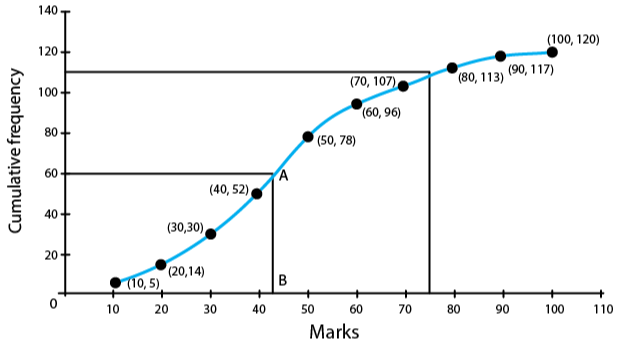(i)  Median = (120 + 1)/ 2 = 60.5th term

Through mark 60.5, draw a parallel line to x-axis which meets the curve at A. From A draw a perpendicular to x-axis meeting it at B.

Then, the value of point B is the median = 43

(ii) Number of students who obtained up to 75% marks in the test = 110

Number of students who obtained more than 75% marks in the test = 120 – 110 = 10

(iii) Number of students who obtained less than 40% marks in the test = 52 (from the graph; x = 40, y = 52)

(iv) Lower quartile = Q1 = 120 x (1/4) = 30th term = 30

8. Using a graph paper, draw an ogive for the following distribution which shows a record of the width in kilograms of 200 students.

 Weight Frequency 40 – 45 5 45 – 50 17 50 – 55 22 55 – 60 45 60 – 65 51 65 – 70 31 70 – 75 20 75 – 80 9

Use your ogive to estimate the following:

(i) The percentage of students weighing 55 kg or more

(ii) The weight above which the heaviest 30% of the student fall

(iii) The number of students who are (a) underweight (b) overweight, if 55.70 kg is considered as standard weight.

Solution:

 Weight Frequency c. f. 40-45 5 5 45-50 17 22 50-55 22 44 55-60 45 89 60-65 51 140 65-70 31 171 70-75 20 191 75-80 9 200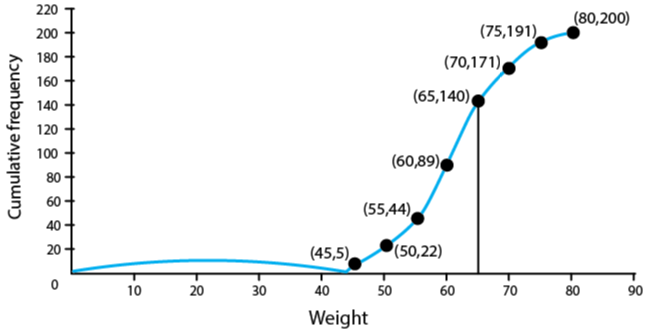(i) The number of students weighing more than 55 kg = 200 – 44 = 156

Thus, the percentage of students weighing 55 kg or more = (156/200) x 100 = 78 %

(ii) 30% of students = (30 x 200)/ 100 = 60

Heaviest 60students in weight = 9 + 21 + 30 = 60

Weight = 65 kg (From table)

(iii) (a) underweight students when 55.70 kg is standard = 46 (approx.) from graph

(b) overweight students when 55.70 kg is standard = 200 – 55.70 = 154 (approx.) from graph

9. The distribution, given below, shows the marks obtained by 25 students in an aptitude test. Find the mean, median and mode of the distribution.

 Marks obtained 5 6 7 8 9 10 No. of students 3 9 6 4 2 1

Solution:

 Marks obtained(x) No. of students (f) c.f. fx 5 3 3 15 6 9 12 54 7 6 18 42 8 4 22 32 9 2 24 18 10 1 25 10 Total 25 171

Number of terms = 25

(i) Mean = 171/25 = 6.84

(ii) Median = (25 + 1)/ 2 th = 13th term = 7

(iii) Mode = 6 since it has the maximum frequency i.e. 6

10. The mean of the following distribution is 52 and the frequency of class interval 30 – 40 is ‘f’. Find f.

 Class Interval 10 – 20 20 – 30 30 – 40 40 – 50 50 – 60 60 – 70 70 – 80 Frequency 5 3 f 7 2 6 13

Solution:

 C.I. Frequency(f) Mid value (x) fx 10-20 5 15 75 20-30 3 25 75 30-40 f 35 35f 40-50 7 45 315 50-60 2 55 110 60-70 6 65 390 70-80 13 75 975 Total 36 + f 1940 + 35f

Mean = ∑ fx/ ∑ f = (1940 + 35f)/ (36 + f) …… (i)

But, given mean = 52 …. (ii)

From (i) and (ii), we have

(1940 + 35f)/ (36 + f) = 52

1940 + 35f = 1872 + 52f

17f = 68

Thus, f = 4

11. The monthly income of a group of 320 employees in a company is given below:

 Monthly Income (thousands) No. of employees 6 – 7 20 7 – 8 45 8 – 9 65 9 – 10 95 10 – 11 60 11 – 12 30 12 – 13 5

Draw an ogive of the given distribution on a graph paper taking 2 cm = Rs 1000 on one axis and 2 cm = 50 employees on the other axis. From the graph determine:

(i) the median wage.

(ii) number of employees whose income is below Rs 8500.

(iii) if salary of a senior employee is above Rs 11,500, find the number of senior employees in the company.

(iv) the upper quartile.

Solution:

 Monthly Income (thousands) No. of employees (f) Cumulative frequency 6-7 20 20 7-8 45 65 8-9 65 130 9-10 95 225 10-11 60 285 11-12 30 315 12-13 5 320 Total 320

Number of employees = 320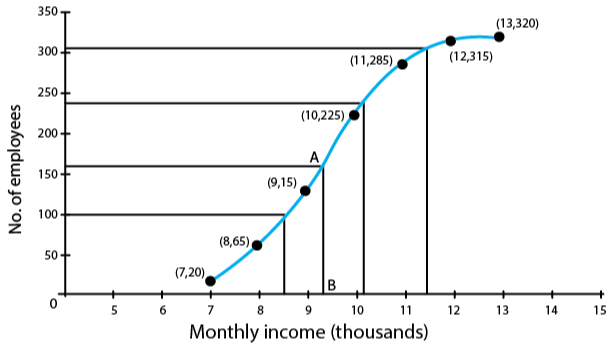(i) Median = 320/2 = 160th term

Through mark 160, draw a parallel line to x-axis which meets the curve at A, From A draw a perpendicular to x-axis meeting it at B.

The value of point B is the median = Rs 9.3 thousands

(ii) The number of employees with income below Rs 8,500 = 95 (approx from the graph)

(iii) Number of employees with income below Rs 11,500 = 305 (approx from the graph)

Thus, the number of employees (senior employees) = 320 – 305 = 15

(iv) Upper quartile = Q3 = 320 x (3/4) = 240th term = 10.3 thousands = Rs 10,300